### Home > MC1 > Chapter 9 > Lesson 9.2.2 > Problem9-73

9-73.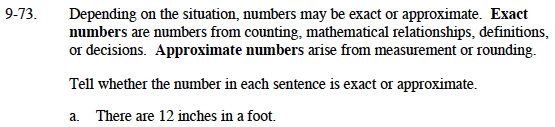Are there exactly 12 inches in a foot, or are there about 12 inches in a foot?

Exact, since this is a mathematical relationship.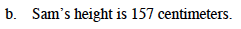Do you think that Sam is exactly 157 centimeters?
Is it possible that he is a few millimeters taller or shorter than this measurement?

Approximate, since this is a measurement.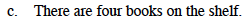Did this number come from counting? If so, it is exact.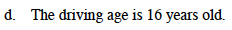The government decided that 16 years old was the correct driving age.
Do you think this is an exact number or an approximate number?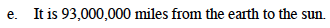Someone had to measure this distance.
Does that make it exact or approximate?

Approximate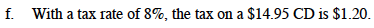Does this look approximate? You can do the work on your own to check if this number is exact.